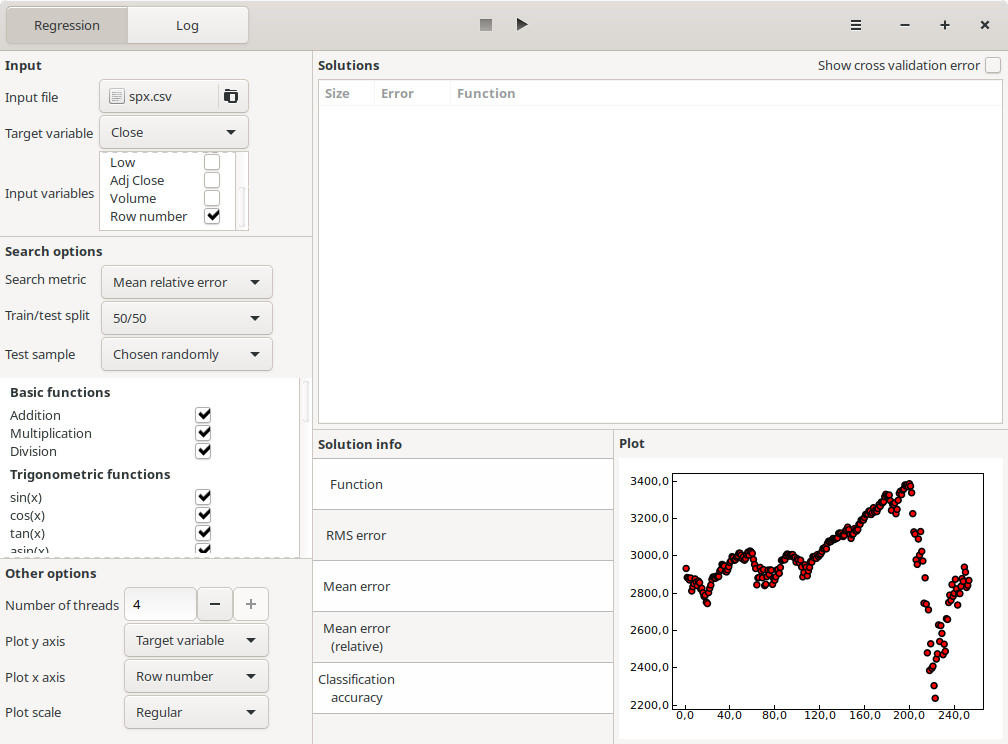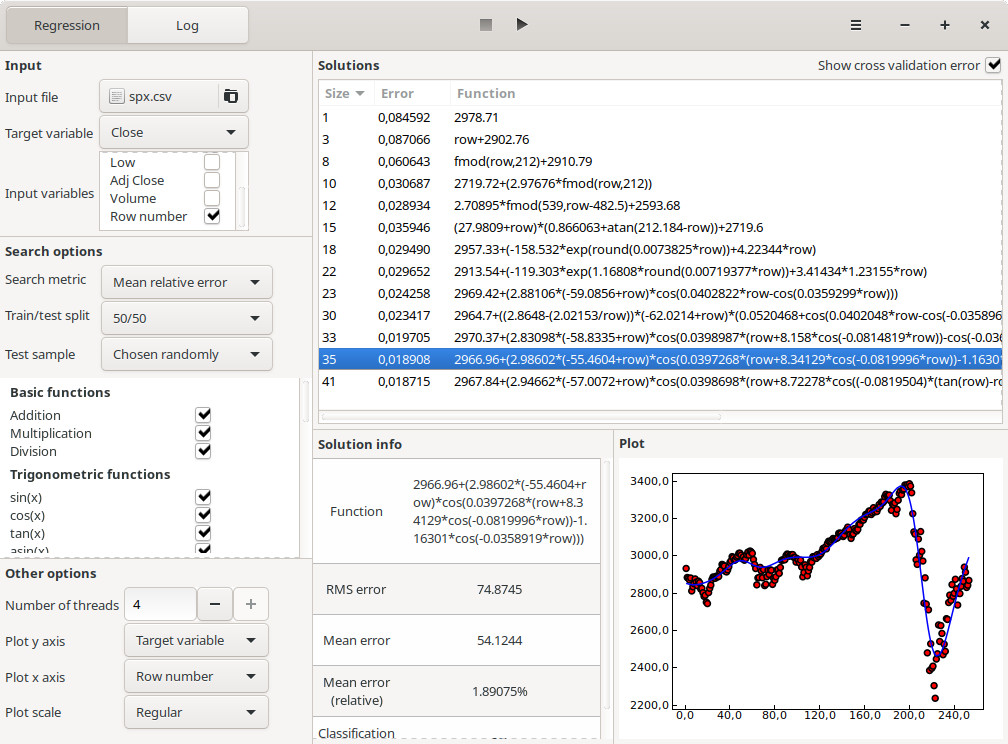A more recent version of this post can be found on our new blog: Using R to visualize a Symbolic Regression model

In this article, we are going to show how a symbolic regression model can be visualized using the R programming language. The model will be generated using the TuringBot software, and we are going to use the ggplot2 library for the visualization.

The dataset that we are going to use consists of the closing prices for the S&P 500 index in the last year, downloaded from Yahoo Finance. The CSV file, which also contains additional columns like open, high, low and volume, can be found here: spx.csv

After opening TuringBot and selecting this file from the menu on the upper left of the interface, we select “Row number” as the input variable and “Close” as the target variable. This way, our model will find the close price as a function of the index of the trading day (1, 2, 3, etc). We will also use a randomly selected 50:50 train/test split to make our model more robust, and “mean relative error” as the optimization metric because we are more interested in the shape of the model than in specific values.

This is what the interface will look like:Clicking on the play button at the top, the optimization is started, using all the CPU cores in the computer for greater performance. The models encountered so far are seen in the “Solutions” box.

After letting the optimization run for a few minutes, we can click on the “Show cross validation error” box on the upper right of the interface to see the out-of-sample performance of each model, and use this information to select the best one, which in this case turned out to be a combination of cosines and multiplications:Now that we have the model, we are going to visualize it using ggplot2. The following script loads the input CSV file and plots it along with the model that we just selected:

library(ggplot2)

data\$idx <- as.numeric(row.names(data))
print(data)

eq = function(row){2966.96+(2.98602*(-55.4604+row)*cos(0.0397268*(row+8.34129*cos(-0.0819996*row))-1.16301*cos(-0.0358919*row)))}

p <- ggplot(data, aes(x=idx, y=Close)) + geom_point()
#p <- ggplot() + geom_line(aes(x=idx, y=Close), data=data)

p + stat_function(fun=eq, color='blue')

#png("test.png")
#print(p)


And this is the final result:This demonstrates the power and simplicity of symbolic regression models: we have managed to readily implement and visualize a deep learning model generated using TuringBot into R, something that would be much harder if the model was a black-box like a neural network or a random forest.